## Double Integrals

In calculus of a single variable the definite integralfor f(x)>=0 is the area under the curve f(x) from x=a to x=b. For general f(x) the definite integral is equal to the area above the x-axis minus the area below the x-axis.

The definite integral can be extended to functions of more than one variable. Consider a function of 2 variables z=f(x,y). The definite integral is denoted bywhere R is the region of integration in the xy-plane.  For positive f(x,y), the definite integral is equal to the volume under the surface z=f(x,y) and above xy-plane for x and y in the region R. This is shown in the figure below.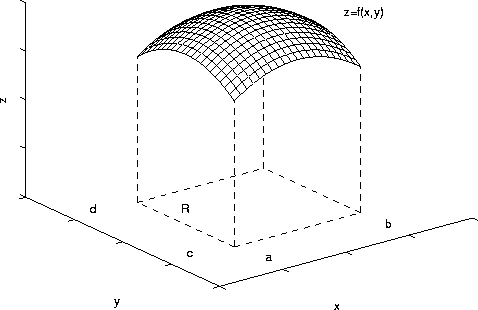For general f(x,y), the definite integral is equal to the volume above the xy-plane minus the volume below the xy-plane. This page includes the following sections:

Double integrals arise in a number of areas of science and engineering, including computations of

• Area of a 2D region
• Volume
• Mass of 2D plates
• Force on a 2D plate
• Average of a function
• Center of Mass and Moment of Inertia
• Surface Area

As in the case of an integral of a function of one variable, a double integral is defined as a limit of a Riemann sum. Suppose we subdivide the region R into subrectangles as in the figure below (say there are M rectangles in the x direction and N rectangles in the y direction).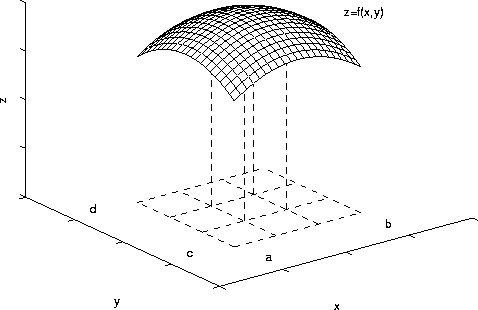Label the rectangles R_ij where 1<=i<=M and 1<=j<=N. Think of the definite integral as representing volume. The volume under the surface above rectangle R_ij is approximately f(x_i,y_j)A_ij, where A_ij is area of the rectangle and f(x_i,y_j) is the approximate height of the surface in the rectangle. Here (x_i,y_j) is some point in the rectangle R_ij. If we sum over all rectangles we have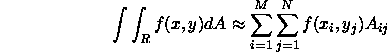In the limit as the size of the rectangles goes to 0, the sum on the right converges to a value which is the definite integral. The quantity f(x,y)dA in the definite integral represents the volume in some infinitesimal region around the point (x,y). The region is so small that the f(x,y) only varies infinitesimally in the region. The double integral sign says: add up volumes in all the small regions in R.

Suppose that f(x,y) is continuous on a rectangular region in the xy plane as shown above. The double integralrepresents the volume under the surface. We can compute the volume by slicing the three-dimensional region like a loaf of bread. Suppose the slices are parallel to the y-axis. An example of slice between x and x+dx is shown in the figure.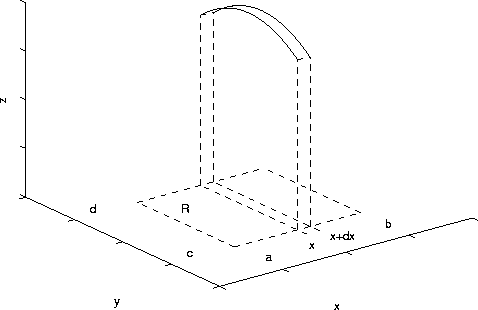In the limit of infinitesimal thickness dx, the volume of the slice is the product of the cross-sectional area and the thickness dx. The cross sectional area is the area under the curve f(x,y) for fixed x and y varying between c and d. (Note that if the thickness dx is infinitesimal, x varies only infinitesimally on the slice. We can assume that x is constant.) The picture below shows the cross-sectional area.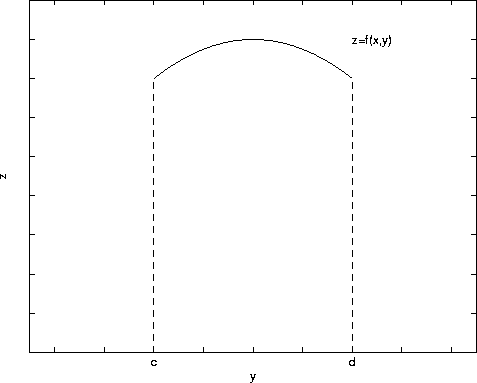The area is given by the integralThe variable of integation is y and x is a CONSTANT. The cross-sectional area depends on x and this is why we write C=C(x). The volume of the slice between x and x+dx is C(x)dx. The total volume is the sum of the volumes of all the slices between x=a and x=b: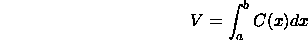If substitute for C(x), we obtain: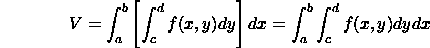This is an example of an iterated integral. One integrates with respect to y first, then x. The integrals with respect to y and x are called the inner and outer integrals, respectively. Alternatively, one can make slices that are parallel to the x-axis. In this case the volume is given byThe inner integral corresponds to the cross-sectional area of a slice between y and y+dy.

The quantities f(x,y)dydx and f(x,y)dxdy represent the value of the double integral in the infinitesimal rectangle between x and x+dx and y and y+dy. The length and width of the rectangle are dx and dy, respectively. Hence dydx (or dxdy) is the area of the rectangle. We can make the connection dA=dydx (or dA=dxdy).

Consider the double integral: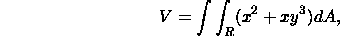where R is the rectangle 0<=x<=1, 1<=y<=2. Suppose we integrate with respect to y first. Then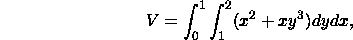The inner integral isNote that we treat x as a constant as we integrate with respect to y. The integral is equal toWe are now left with the integral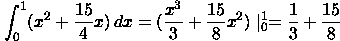Alternatively, we can integrate with respect to x first and then y. We haveThe inner integral is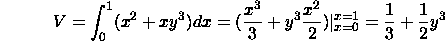Note that we treat y as a constant as we integrate with respect to x. The outer integral isThe two approaches yield the same answer.

In applications, integrals like the one above arise in computations of

• Area: if f(x,y)=1, then the double integrals gives the area of region R.
• Volume: the integral is equal to volume under the surface z=f(x,y) above the region R.
• Mass: if R is a plate and f(x,y) is density per unit area of the plate, then the integral is equal to the mass of the plate.
• Force: if f(x,y) is the force per unit area on the plate in the downward direction, then integral is the total force on the plate.
• Average: the integral divided by the area of the region R is the the average of the function f(x,y) on R.

In this section we consider double integrals over more general regions. Suppose that the region R is defined by G_1(x)<=y<=G_2(x) with a<=x<=b. This is called a vertically simple region. The double integral is given byTo derive this formula we slice the three-dimensional region into slices parallel to the y-axis. The figure below shows a top view of slice between x and x+dx.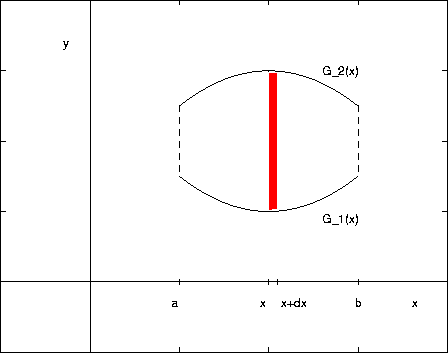The inner integral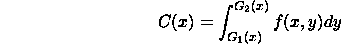is the cross-sectional area of the slice between x and x+dx. The volume of the slice between x and x+dx is C(x)dx. The total volume is the sum of the volumes of all the slices: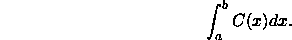If R is the horizontally simple region - that is, if the region is defined by c<=y<=d and H_1(y)<=x<=H_2(y), then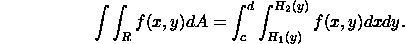In this case the slices are parallel to the x-axis. The inner integralis the cross-sectional area of the slice between y and y+dy. The volume of the slice between y and y+dy is C(y)dy. The total volume isIn some cases a region may be neither vertically nor horizontally simple. However, in general, a region can be split up into vertically and horizontally simple regions.

Compute the double integral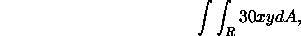where R is bounded by y=x and y=x^2. See figure below.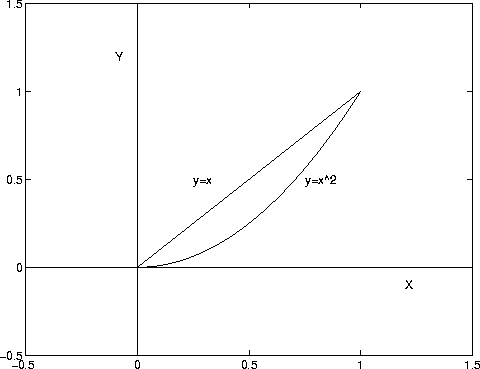We can treat the region R as a vertically simple region. In this case the integral is given by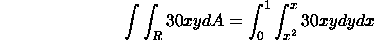The inner integral is (remember x is a constant)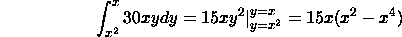The outer integral is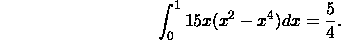One can also treat the region as a horizontally simple region. The left hand function y=x can be written x=H_1(y)=y. The right hand function y=x^2 can be written x=sqrt(y). The iterated integral is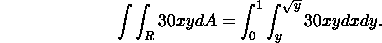You should check that the answer is 5/4.

Copyright © 1996 Department of Mathematics, Oregon State University

If you have questions or comments, don't hestitate to contact us.